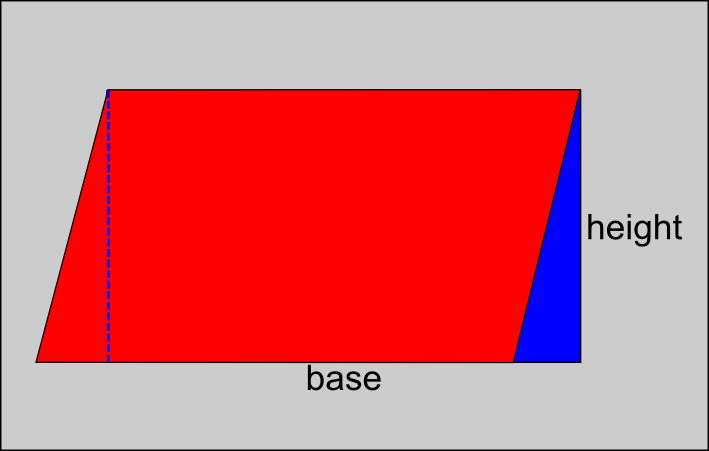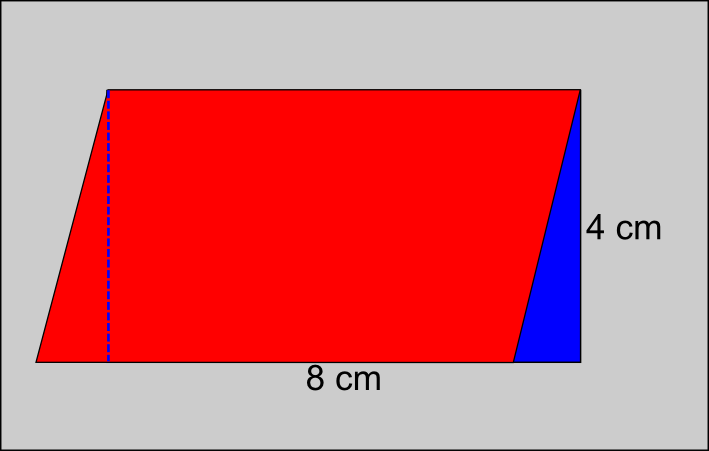The Singing Hedgehog Guide to:
Area - Parallelograms
(and Rhombuses)

The area of any parallelogram is the same as a rectangle of the same length.
You can use this to find the area of a rhombus, if you have the right dimensions.First we draw a rectangle the same length as the parallelogram.
We can see that the small red triangle on the left will exactly fit over
the blue triangle on the right.
Obviously the area of the parallelogram is equal to the area of the rectangle.

To work out the area of a parallelogram we use a simple formula:

area = base x perpendicular height

Notice that the height is the perpendicular [at right angles]
distance from the base to the top and not one of the sides.

So for this parallelogram:area = 8 x 4 = 32 cm²

next page